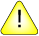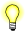This version of this document is no longer maintained. For the latest documentation, see http://www.qnx.com/developers/docs.

# cos(), cosf()

Compute the cosine of an angle

## Synopsis:

```#include <math.h>

double cos( double x );

float cosf( float x );```

## Arguments:

x
The angle, in radians, for which you want to compute the cosine.

## Library:

libm

Use the -l m option to qcc to link against this library.

## Description:

These functions compute the cosine of x (specified in radians).An argument with a large magnitude may yield results with little or no significance.

## Returns:

The cosine of x.If an error occurs, these functions return 0, but this is also a valid mathematical result. If you want to check for errors, set errno to 0, call the function, and then check errno again. These functions don't change errno if no errors occurred.

## Examples:

```#include <math.h>
#include <stdio.h>
#include <stdlib.h>

int main( void )
{
double value;

value = cos( M_PI );
printf( "value = %f\n", value );

return EXIT_SUCCESS;
}```

produces the output:

`value = -1.000000`

## Classification:

Safety:
Cancellation point No
Interrupt handler No
Signal handler No